The Flower of Life
or
Seven Circles Make a Hexagon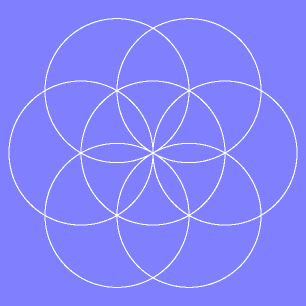The design above is called the Flower of Life and is constructed with 7 circles of equal size. To make this design draw a circle with a compass, then place the point of the compass on the edge of the circle you drew and draw another circle. Next move the point to one of the intersections of the two circles and draw another circle. Do this at each point a circle crosses the first circle. 6 circles neatly fit around the first. If the intersections with the center circle are connected, a hexagon is formed which is made up of 6 eqilateral triangles.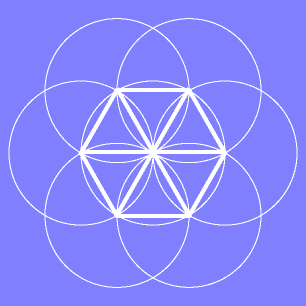Connect the outer intersections and two opposing egilateral triangles are formed making a six pointed star.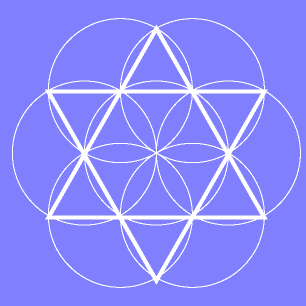The angles created in these shapes are 60° and 120°. If the raduis of the original circle is 1 then the perimiter of the hexagon is 6. The distance from one point to another is either 1,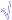3, or 2 (the diameter).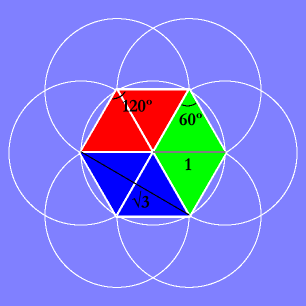Without connecting the intersections two types of rounded shapes are formed by this design. One is a large leaf shape and the other is a smaller petal shape.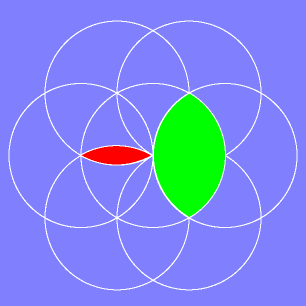Back